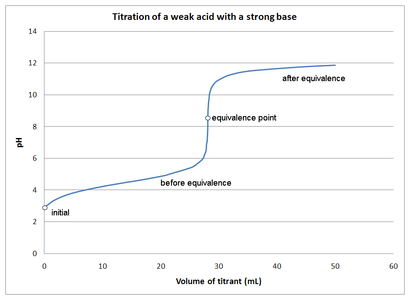## Weak Acid-Strong Base Titrations

#### Learning Objective

• Distinguish a weak acid-strong base titration from other types of titrations.

#### Key Points

• In an acid-base titration, the titration curve reflects the strengths of the corresponding acid and base.
• If one reagent is a weak acid or base and the other is a strong acid or base, the titration curve is irregular, and the pH shifts less with small additions of titrant near the equivalence point.
• Acid-base titrations depend on the neutralization between an acid and a base when mixed in solution.
• The endpoint and the equivalence point are not exactly the same: the equivalence point is determined by the stoichiometry of the reaction, while the endpoint is just the color change from the indicator.

#### Terms

• stoichiometryThe study and calculation of quantitative (measurable) relationships of the reactants and products in chemical reactions (chemical equations).
• bufferA solution used to stabilize the pH (acidity) of a liquid.
• pHThe negative of the logarithm to base 10 of the concentration of hydrogen ions, measured in moles per liter; a measure of acidity or alkalinity of a substance, which takes numerical values from 0 (maximum acidity) through 7 (neutral) to 14 (maximum alkalinity).
• equivalence pointThe point in a chemical reaction at which chemically equivalent quantities of acid and base have been mixed.

Titrations are reactions between specifically selected reactants—in this case, a strong base and a weak acid. A titration curve reflects the strength of the corresponding acid and base, showing the pH change during titration. The titration curve demonstrating the pH change during the titration of the strong base with a weak acid shows that at the beginning, the pH changes very slowly and gradually. This indicates the formation of a buffer system as the titration approaches the equivalence point.

At the equivalence point and beyond, the curve is typical of a titration of, for example, NaOH and HCl. When the NaOH is in excess, the pH change is the same as in any system dominated by NaOH.Titration of a weak Acid with a strong baseThis figure depicts the pH changes during a titration of a weak acid with a strong base.

The initial pH of the solution at the beginning of the titration is approximately that of the weak acid in water. At the equivalence point, all of the weak acid is neutralized and converted to its conjugate base (the number of moles of H+ = added number of moles of OH). However, the pH at the equivalence point does not equal 7. This is due to the production of conjugate base during the titration. The resulting solution is slightly basic. The endpoint and the equivalence point are not exactly the same: the equivalence point is determined by the stoichiometry of the reaction, while the endpoint is just the color change from the indicator.

## Example:

The titration of acetic acid (HC2H3O2) with NaOH.

$HC_2H_3O_2 + OH^- \rightarrow H_2O + C_2H_3O_2^-$

During this titration, as the OH reacts with the H+ from acetic acid, the acetate ion (C2H3O2) is formed. This conjugate base reacts with water to form a slightly basic solution.

$C_2H_3O_2^- + H_2O \rightarrow HC_2H_3O_2 + OH^-$